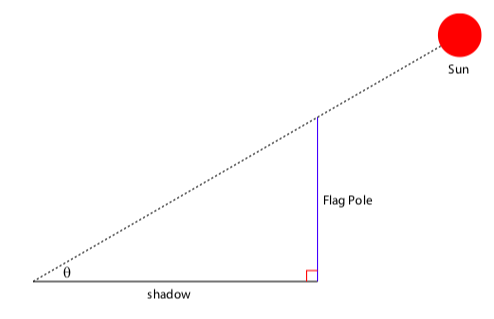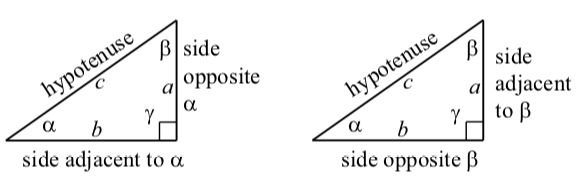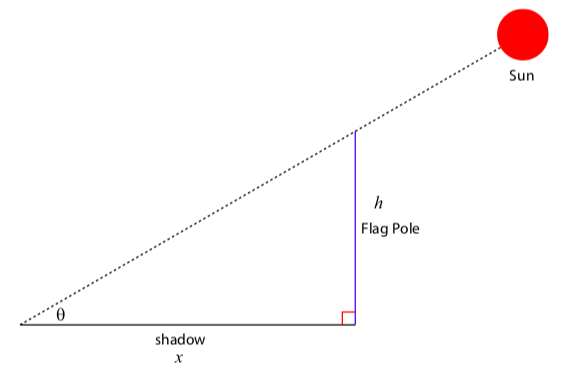$$\newcommand{\id}{\mathrm{id}}$$ $$\newcommand{\Span}{\mathrm{span}}$$ $$\newcommand{\kernel}{\mathrm{null}\,}$$ $$\newcommand{\range}{\mathrm{range}\,}$$ $$\newcommand{\RealPart}{\mathrm{Re}}$$ $$\newcommand{\ImaginaryPart}{\mathrm{Im}}$$ $$\newcommand{\Argument}{\mathrm{Arg}}$$ $$\newcommand{\norm}{\| #1 \|}$$ $$\newcommand{\inner}{\langle #1, #2 \rangle}$$ $$\newcommand{\Span}{\mathrm{span}}$$

3.2: Right Triangles

$$\newcommand{\vecs}{\overset { \rightharpoonup} {\mathbf{#1}} }$$

$$\newcommand{\vecd}{\overset{-\!-\!\rightharpoonup}{\vphantom{a}\smash {#1}}}$$

Focus Questions

The following questions are meant to guide our study of the material in this section. After studying this section, we should understand the concepts mo- tivated by these questions and be able to write precise, coherent answers to these questions.

• How does the cosine relate sides and acute angles in a right triangle? Why?
• How does the sine relate sides and acute angles in a right triangle? Why?
• How does the tangent relate sides and acute angles in a right triangle? Why?
• How can we use the cosine, sine, and tangent of an angle in a right triangle to help determine unknown parts of that triangle?

Beginning Activity

The following diagram shows a typical right triangle. The lengths of the three sides of the right triangle are labeled as $$a$$, $$b$$, and $$c$$. The angles opposite the sides of lengths $$a$$, $$b$$, and $$c$$ are labeled $$\alpha$$ (alpha), $$\beta$$ (beta), and $$\gamma$$ (gamma), respectively. (Alpha, beta, and gamma are the first three letters in the Greek alphabet.) The small square with the angle $$\gamma$$ indicates that this is the right angle in the right triangle. The triangle, of course, has three sides. We call the side opposite the right angle (the side of length $$c$$ in the diagram) the hypotenuse of the right triangle.

When we work with triangles, the angles are usually measured in degrees and so we would say that $$\gamma$$ is an angle of $$90^\circ$$.1. What can we conclude about $$a$$, $$b$$, and $$c$$ from the Pythagorean Theorem? When working with triangles, we usually measure angles in degrees. For the fractional part of the degree measure of an angle, we often used decimals but we also frequently use minutes and seconds.
2. What is the sum of the angles in a triangle? In this case, what is $$\alpha + \beta + \gamma$$?
3. What is the sum of the two acute angles in a right triangle. In this case, what is $$\alpha + \beta$$?
4. How many minutes are in a degree? How many seconds are in a minute?
5. Determine the solution of the equation $$7.3 = \dfrac{118.8}{x}$$ correct to the nearest thousandth. (You should be able to show that $$x \approx 2.575$$.)
6. Determine the solution of the equation $$(\sin^\circ) = \dfrac{5}{x}$$ correct to the nearest ten-thousandth. (You should be able to show that $$x \approx 9.4354$$.)

Suppose you want to find the height of a tall object such as a flagpole (or a tree or a building). It might be inconvenient (or even dangerous) to climb the flagpole and measure it, so what can you do? It might be easy to measure the length the shadow the flagpole casts and also the angle $$\theta$$ determined by the ground level to the sun (called the angle of elevation of the object) as in Figure $$\PageIndex{1}$$. In this section, we will learn how to use the trigonometric functions to relate lengths of sides to angles in right triangles and solve this problem as well as many others.Figure $$\PageIndex{1}$$: Finding the height of a flagpole (drawing not to scale)

Trigonometric Functions and Right Triangles

We have seen how we determine the values of the trigonometric functions of an angle $$\theta$$ by placing $$\theta$$ in standard position and letting $$(x, y)$$ be the point of intersection of the terminal side of angle $$\theta$$ with a circle of radius $$r$$. Then

1. $$\cos(\theta) = \dfrac{x}{r}$$
2. $$\sin(\theta) = \dfrac{y}{r}$$
3. $$\tan(\theta) = \dfrac{y}{x}$$ if $$x \neq 0$$
4. $$\sec(\theta) = \dfrac{r}{x}$$ if $$x \neq 0$$
5. $$\csc(\theta) = \dfrac{r}{y}$$ if $$y \neq 0$$
6. $$\cot(\theta) = \dfrac{x}{y}$$ if $$y \neq 0$$

In our work with right triangles, we will use only the sine, cosine, and tangent functions.

Now we will see how to relate the trigonometric functions to angles in right triangles. Suppose we have a right triangle with sides of length $$x$$ and $$y$$ and hypotenuse of length $$r$$. Let $$\theta$$ be the angle opposite the side of length $$y$$ as shown in Figure $$\PageIndex{2}$$. We can now place our triangle such that the angle $$\theta$$ is in standard position in the plane and the triangle will fit into the circle of radius $$r$$ as shown at right in Figure $$\PageIndex{3}$$.

By the definition of our trigonometric functions we then have

$\cos(\theta) = \dfrac{x}{r}$

$\sin(\theta) = \dfrac{y}{r}$

$\tan(\theta) = \dfrac{y}{x}$

If instead of using $$x$$, $$y$$, and $$r$$, we label $$y$$ as the length of the side opposite the acute angle $$\theta$$, $$x$$ as the length of the side adjacent to the acute angle $$\theta$$, and $$r$$ as the length of the hypotenuse, we get the following figure:Figure $$\PageIndex{2}$$: A right triangleFigure $$\PageIndex{3}$$: Right triangle in standard positionSo we see that

$\sin(\theta) = \dfrac{length \space of \space side \space opposite\space \theta}{length \space of \space hypotenuse} \space\space \sin(\theta) = \dfrac{opposite}{hypotenuse}$

$\cos(\theta) = \dfrac{length \space of \space side \space adjacent\space to \space \theta}{length \space of \space hypotenuse} \space\space \cos(\theta) = \dfrac{adjacent}{hypotenuse}$

$\tan(\theta) = \dfrac{length \space of \space side \space opposite\space \theta}{length \space of \space side \space adjacent\space to \space \theta} \space\space \tan(\theta) = \dfrac{opposite}{adjacent}$

The equations on the right are convenient abbreviations of the correct equations on the left.

Exercise $$\PageIndex{1}$$

We must be careful when we use the terms opposite and adjacent because the meaning of these terms depends on the angle we are using. Use the diagrams in Figure 3.8 to determine formulas for each of the following in terms of a, b, and c.Figure $$\PageIndex{4}$$: Labels for a right triangle

1. $$\cos(\alpha) = ?$$
2. $$\sin(\alpha) = ?$$
3. $$\tan(\alpha) = ?$$
4. $$\cos(\beta) = ?$$
5. $$\sin(\beta) = ?$$
6. $$\tan(\beta) = ?$$

We should also note that with the labeling of the right triangle shown in Figure $$\PageIndex{4}$$, we can use the Pythagorean Theorem and the fact that the sum of the angles of a triangle is $$180$$ degrees to conclude that $a^{2} + b^{2} = c^{2}$ and $\alpha + \beta + \gamma = 180^\circ$ $\gamma = 90^\circ$ $\alpha + \beta = 90^\circ$

Example $$\PageIndex{1}$$

Suppose that one of the acute angles of a right triangle has a measure of $$35^\circ$$ and that the side adjacent to this angle is 8 inches long. Determine the other acute angle of the right triangle and the lengths of the other two sides.

Solution

The first thing we do is draw a picture of the triangle. (The picture does not have to be perfect but it should reasonably reflect the given information.) In making the diagram, we should also label the unknown parts of the triangle. One way to do this is shown in the diagram.One thing we notice is that $$35^\circ + \theta = 90^\circ$$ and $$\theta = 55^\circ$$. We can also use the cosine and tangent of $$35^\circ$$ to determine the values of $$a$$ and $$c$$.

\begin{align*} \cos(35^\circ) &= \dfrac{8}{c} \\[4pt] c\cos(35^\circ) &= 8 \\[4pt] c &= \dfrac{8}{\cos(35^\circ)} \\[4pt] c &\approx 9.76620 \end{align*}

\begin{align*} \tan(35^\circ) &= \dfrac{a}{8} \\[4pt] 8\tan(35^\circ) &= a \\[4pt] a &\approx 5.60166 \end{align*}

Before saying that this example is complete, we should check our results. One way to do this is to verify that the lengths of the three sides of the right triangle satisfy the formula for the Pythagorean Theorem. Using the given value for one side and the calculated values of $$a$$ and $$c$$, we see that

$8^{2} + a^{2} \approx 95.379$ $c^{2} \approx 95.379 \nonumber$

So we see that our work checks with the Pythagorean Theorem.

Solving Right Triangles

What we did in Example $$\PageIndex{1}$$ is what is called solving a right triangle. Please note that this phrase is misleading because you cannot really “solve” a triangle. Unfortunately, this phrase is ensconced in the vernacular of trigonometry and so we will continue to use it. The idea is that if we are given enough information about the lengths of sides and measures of angles in a right triangle, then we can determine all of the other values. The next progress check is also an example of “solving a right triangle.”

Exercise $$\PageIndex{2}$$

The length of the hypotenuse of a right triangle is $$17$$ feet and the length of one side of this right triangle is $$5$$ feet. Determine the length of the other side and the two acute angles for this right triangle.

Hint: Draw a picture and label the third side of the right triangle with a variable and label the two acute angles as $$\alpha$$ and $$\beta$$.

We let $$\alpha$$ be the angle opposite the side of length $$5$$ feet and let $$\beta$$ be the angle adjacent to that side. We then see that

$\sin(\alpha) = \dfrac{5}{17}$ $\alpha = \arcsin(\dfrac{5}{17})$ $\alpha \approx 17.1046^\circ$

$\cos(\beta) = \dfrac{5}{17}$ $\beta = \arccos(\dfrac{5}{17})$ $\beta \approx 72.8954^\circ$

As a check, we notice that $$\alpha + \beta = 90^\circ$$. We can use the Pythagorean theorem to determine the third side, which using our notation, is $$b$$. So $5^{2} + b^{2} = 17^{2}$

and so we see that $$b = \sqrt{264} \approx 16.2481$$ feet.

Applications of Right Triangles

As the examples have illustrated up to this point, when working on problems involving right triangles (including application problems), we should:

• Draw a diagram for the problem.
• Identify the things you know about the situation. If appropriate, include this information in your picture.
• Identify the quantity that needs to be determined and give this quantity a variable name. If appropriate, include this information in your diagram.
• Find an equation that relates what is known to what must be determined. This will often involve a trigonometric function or the Pythagorean Theorem.
• Solve the equation for the unknown. Then think about this solution to make sure it makes sense in the context of the problem.
• If possible, find a way to check the result.

We return to the example given in the introduction to this section on page 179. In this example, we used the term angle of elevation. This is a common term (as well as angle of depression) in problems involving triangles. We can define an angle of elevation of an object to be an angle whose initial side is horizontal and has a rotation so that the terminal side is above the horizontal. An angle of depression is then an angle whose initial side is horizontal and has a rotation so that the terminal side is below the horizontal (Figure 3.9).Figure $$\PageIndex{5}$$: Angle of Elevation and Angle of Depression

Example $$\PageIndex{2}$$: Determining the Height of a Flagpole

Suppose that we want to determine the height of a flagpole and cannot measure the height directly. Suppose that we measure the length of the shadow of the flagpole to be $$44$$ feet, $$5$$ inches. In addition, we measure the angle of elevation of the sun to be $$33^\circ15'$$.

The first thing we do is to draw the diagram. In the diagram, we let $$h$$Figure $$\PageIndex{6}$$: Finding the height of a flagpole (drawing not to scale)

be the height of the flagpole, $$x$$ be the length of the shadow, and $$\theta$$ be the angle of elevation. We are given values for $$x$$ and $$\theta$$, and we see that

$\tan(\theta) = \dfrac{h}{x}$ $x\tan(\theta) = h$

So we can now determine the value of $$h$$, but we must be careful to use a decimal (or fractional) value for $$x$$ (equivalent to 44 feet, 5 inches) and a decimal (or fractional) value for $$\theta$$ (equivalent to $$33^\circ15'$$). So we will use $x = 44 + \dfrac{5}{12}$ and $\theta = (33 + \dfrac{15}{60})^\circ$

Using this and equation (1), we see that $h = (44 + \dfrac{5}{12})\tan(33 + \dfrac{15}{60})^\circ$ $h \approx 29.1208 \space feet$

The height of the flagpole is about $$29.12$$ feet or $$29$$ feet, $$1.4$$ inches.

Exercise $$\PageIndex{3}$$

A company needs to build a wheelchair accessible ramp to its entrance. The Americans with Disabilities Act Guidelines for Buildings and Facilities for ramps state the “The maximum slope of a ramp in new construction shall be $$1:12$$.”

1. The $$1:12$$ guideline means that for every 1 foot of rise in the ramp there must be $$12$$ feet of run. What is the angle of elevation (in degrees) of such a ramp?
2. If the company’s entrance is $$7.5$$ feet above the level ground, use trigonometry to approximate the length of the ramp that the company will need to build using the maximum slope. Explain your process.

With a rise of $$1$$ foot for every $$12$$ feet of run, we see if we let $$\theta$$ be the angle of elevation, then

$\tan(\theta) = \dfrac{1}{12}$

$\theta = \arctan(\dfrac{1}{12})$

$\theta \approx 4.7636^\circ$

The length of the ramp will be the hypotenuse of the right triangle. So if we let $$h$$ be the length of the hypotenuse, then

$\sin(\theta) = \dfrac{7.5}{h}$

$h = \dfrac{7.5}{\sin(\theta)}$

$h \approx 90.3120$

The length of the hypotenuse is approximately $$90.3$$ feet. We can check our result by determining the length of the third side, which is $$7.5 \cdot 12$$ or $$90$$ feet and then verifying the result of the Pythagorean theorem. We can verify that

$7.5^{2} + 90^{2} \approx 90.3120^{2}.$

Exercise $$\PageIndex{4}$$

This is a variation of Example 3.19. Suppose that the flagpole sits on top a hill and that we cannot directly measure the length of the shadow of the flagpole as shown in Figure 3.19.

Some quantities have been labeled in the diagram. Angles $$\alpha$$ and $$\beta$$ are angles of elevation to the top of the flagpole from two different points on level ground. These points are $$d$$ feet apart and directly in line with the flagpole. The problemFigure $$\PageIndex{7}$$: Flagpole on a hill

is to determine $$h$$, the height from level ground to the top of the flagpole. The following measurements have been recorded.

$\alpha = 43.2^\circ$ $\beta = 34.7^\circ$ $d = 22.75 feet$

Notice that a value for $$x$$ was not given because it is the distance from the first point to an imaginary point directly below the flagpole and even with level ground.

Please keep in mind that it is probably easier to write formulas in terms of $$\alpha$$, $$\beta$$, and $$\gamma$$ and wait until the end to use the numerical values. For example, we see that $\tan(\alpha) = \dfrac{h}{x}$ and $\tan(\beta) = \dfrac{h}{d + x}$

In equation (1), notice that we know the value of $$\alpha$$. This means if we can determine a value for either $$x$$ or $$h$$, we can use equation (1) to determine the value of the other. We will first determine the value of $$x$$.

1. Solve equation (1) for h and then substitute this into equation (2). Call this equation (3).

2. One of the terms in equation (3) has a denominator. Multiply both sides of equation (3) by this denominator.

3. Now solve the resulting equation for $$x$$ (in terms of $$\alpha$$, $$\beta$$, and $$\gamma$$).

4. Substitute the given values for $$\alpha$$, $$\beta$$, and $$\gamma$$ to determine the value of $$x$$ and

then use this value and equation (1) to determine the value of $$h$$.

5. Is there a way to check to make sure the result is correct?

1. $$h = x\tan(\alpha)$$. So $\tan(\beta) = \dfrac{x\tan(\alpha)}{d + x}$

2. $$\tan(\beta)(d + x) = x\tan(\alpha)$$

3. We can proceed to solve for x as follows:

$d\tan(\beta) + x\tan(\beta) = x\tan(\alpha)$

$d\tan(\beta) = x\tan(\alpha) - x\tan(\beta)$

$d\tan(\beta) = x(\tan(\alpha) - \tan(\beta))$

$\dfrac{d\tan(\beta)}{\tan(\alpha) - \tan(\beta)} = x$

So we see that $$x = \dfrac{22.75\tan(34.7^\circ)}{\tan(43.2^\circ) - \tan(34.7^\circ)} \approx 63.872$$. Using this value $$x$$ we obtain $$h = x\tan(43.2^\circ) \approx 59.980$$. So the top of the flagpole is about $$59.98$$ feet above the ground.

4. There are several ways to check this result. One is to use the values for $$d, h$$, and $$x$$ and the inverse tangent function to determine the values for $$\alpha$$ and $$\beta$$. If we use approximate values for $$d, h$$, and $$x$$, these checks may not be exact. For example,

$\alpha = \arctan(\dfrac{h}{x}) \approx \arctan(\dfrac{59.98}{63.872}) \approx 43.2^\circ$

$\beta = \arctan(\dfrac{h}{d + x}) \approx \arctan(\dfrac{59.980}{22.75 + 63.872}) \approx 34.7^\circ$

Another method to check the results is to use the sine of $$\alpha$$ or $$\beta$$ to determine the length of the hypotenuse of one of the right triangles and then check using the Pythagorean Theorem.

Summary

In this section, we studied the following important concepts and ideas:

Given enough information about the lengths of sides and measures of angles in a right triangle, we can determine all of the other values using the following relationships:$\sin(\theta) = \dfrac{opposite}{hypotenuse}$

$\cos(\theta) = \dfrac{adjacent}{hypotenuse}$

$\tan(\theta) = \dfrac{opposite}{adjacent}$